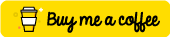# 3x3-equation-solver

Solves systems of 3 equations with three unknowns.

## Usage no npm install needed!

``````<script type="module">
import x3EquationSolver from 'https://cdn.skypack.dev/3x3-equation-solver';
</script>``````

# 3x3-equation-solverSolves systems of 3 equations with three unknowns.

## :cloud: Installation

``````# Using npm
npm install --save 3x3-equation-solver

# Using yarn
``````

## :clipboard: Example

``````// Dependencies
var Solver = require("3x3-equation-solver");

// 3a + b - 5c = 5
// 4a + 2b + 7c = 19
// 5a - 4b + c = 6
console.log(Solver([
[3,  1, -5, 5 ]
, [4,  2,  7, 19]
, [5, -4,  1, 6 ]
], true));
// =>
// { result: [ 2.442231075697211, 1.7569721115537849, 0.8167330677290837 ],
//   results: [ 5, 19, 6 ],
//   coefficients: [ [ 3, 1, -5 ], [ 4, 2, 7 ], [ 5, -4, 1 ] ],
//   determinant: 251,
//   determinants: [ 613, 441, 205 ],
//   solved: true }
``````

## :question: Get Help

There are few ways to get help:

1. Please post questions on Stack Overflow. You can open issues with questions, as long you add a link to your Stack Overflow question.
2. For bug reports and feature requests, open issues. :bug:
3. For direct and quick help, you can use Codementor. :rocket:

## :memo: Documentation

### `Solver(input, raw)`

Solves the system of equations.

#### Params

• Array `input`: An array of arrays containing the coeficients and the results. Example:
``````[
3,  1, -5, 5
, 4,  2,  7, 19
, 5, -4,  1, 6
]
``````
• Boolean `raw`: If it is true, the return value will be an object like described below. Otherwise, the result array will be returned.

#### Return

• Object|Array The actual result (if `raw` is not true) or an object containing the following fields:
• `result` (Array): An array of three values containing the unknown values.
• `coefficients` (Array): An array of arrays containing the coefficients.
• `results` (Array): The equation results.
• `determinant` (Number): The determinant value.
• `determinants` (Array): The unknown determinants.
• `solved` (Boolean): If `true`, that means the system has a solution, otherwise.

## :yum: How to contribute

Have an idea? Found a bug? See how to contribute.

## :sparkling_heart: Support my projects

I open-source almost everything I can, and I try to reply to everyone needing help using these projects. Obviously, this takes time. You can integrate and use these projects in your applications for free! You can even change the source code and redistribute (even resell it).

However, if you get some profit from this or just want to encourage me to continue creating stuff, there are few ways you can do it:

Thanks! :heart:

## :dizzy: Where is this library used?

If you are using this library in one of your projects, add it in this list. :sparkles:

• `no-one-left-behind`